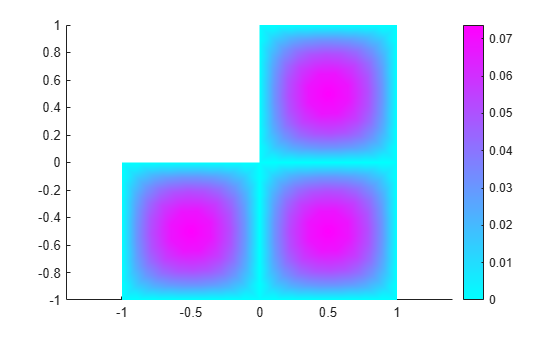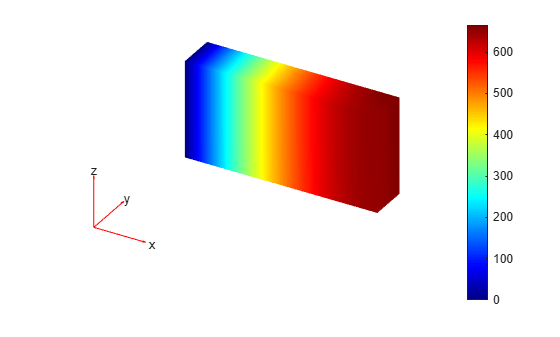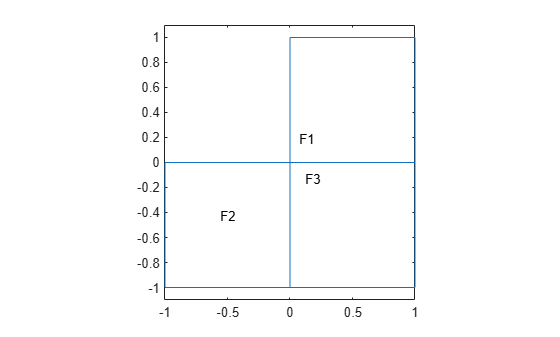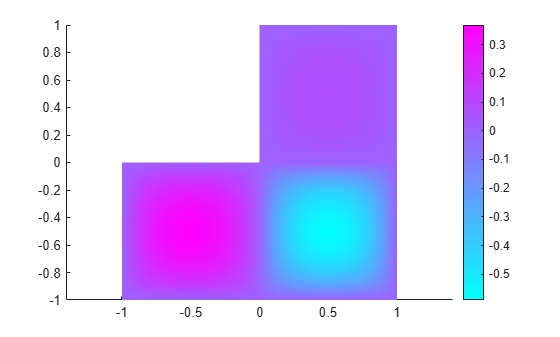specifyCoefficients

Specify coefficients in a PDE model

Description

Coefficients of a PDE

solvepde solves PDEs of the form

$m\frac{{\partial }^{2}u}{\partial {t}^{2}}+d\frac{\partial u}{\partial t}-\nabla ·\left(c\nabla u\right)+au=f$

solvepdeeig solves PDE eigenvalue problems of the form

$\begin{array}{l}-\nabla ·\left(c\nabla u\right)+au=\lambda du\\ \text{or}\\ -\nabla ·\left(c\nabla u\right)+au={\lambda }^{2}mu\end{array}$

specifyCoefficients defines the coefficients m, d, c, a, and f in the PDE model.

example

specifyCoefficients(model,Name,Value) defines the specified coefficients in each Name to each associated Value, and includes them in model. You must specify all of these names: m, d, c, a, and f. This syntax applies coefficients to the entire geometry.

Note

Include geometry in model before using specifyCoefficients.

example

specifyCoefficients(model,Name,Value,RegionType,RegionID) assigns coefficients for a specified geometry region.

example

CA = specifyCoefficients(___) returns a handle to the coefficient assignment object in model.

Examples

collapse all

Specify the coefficients for Poisson's equation $-\nabla \cdot \nabla u=1$.

solvepde addresses equations of the form

$m\frac{{\partial }^{2}u}{\partial {t}^{2}}+d\frac{\partial u}{\partial t}-\nabla \cdot \left(c\nabla u\right)+au=f$.

Therefore, the coefficients for Poisson's equation are $m=0$, $d=0$, $c=1$, $a=0$, $f=1$. Include these coefficients in a PDE model of the L-shaped membrane.

model = createpde();
geometryFromEdges(model,@lshapeg);
specifyCoefficients(model,'m',0,...
'd',0,...
'c',1,...
'a',0,...
'f',1);

Specify zero Dirichlet boundary conditions, mesh the model, and solve the PDE.

applyBoundaryCondition(model,'dirichlet', ...
'Edge',1:model.Geometry.NumEdges, ...
'u',0);
generateMesh(model,'Hmax',0.25);
results = solvepde(model);

View the solution.

pdeplot(model,'XYData',results.NodalSolution)Specify coefficients for Poisson's equation in 3-D with a nonconstant source term, and obtain the coefficient object.

The equation coefficients are $m=0$, $d=0$, $c=1$, $a=0$. For the nonconstant source term, take $f={y}^{2}\mathrm{tanh}\left(z\right)/1000$.

f = @(location,state)location.y.^2.*tanh(location.z)/1000;

Set the coefficients in a 3-D rectangular block geometry.

model = createpde();
importGeometry(model,'Block.stl');
CA = specifyCoefficients(model,'m',0,...
'd',0,...
'c',1,...
'a',0,...
'f',f)
CA =
CoefficientAssignment with properties:

RegionType: 'cell'
RegionID: 1
m: 0
d: 0
c: 1
a: 0
f: @(location,state)location.y.^2.*tanh(location.z)/1000

Set zero Dirichlet conditions on face 1, mesh the geometry, and solve the PDE.

applyBoundaryCondition(model,'dirichlet','Face',1,'u',0);
generateMesh(model);
results = solvepde(model);

View the solution on the surface.

pdeplot3D(model,'ColorMapData',results.NodalSolution)Create a scalar PDE model with the L-shaped membrane as the geometry. Plot the geometry and subdomain labels.

model = createpde();
geometryFromEdges(model,@lshapeg);
pdegplot(model,'FaceLabels','on')
axis equal
ylim([-1.1,1.1])Set the c coefficient to 1 in all domains, but the f coefficient to 1 in subdomain 1, 5 in subdomain 2, and -8 in subdomain 3. Set all other coefficients to 0.

specifyCoefficients(model,'m',0,'d',0,'c',1,'a',0,'f',1,'Face',1);
specifyCoefficients(model,'m',0,'d',0,'c',1,'a',0,'f',5,'Face',2);
specifyCoefficients(model,'m',0,'d',0,'c',1,'a',0,'f',-8,'Face',3);

Set zero Dirichlet boundary conditions to all edges. Create a mesh, solve the PDE, and plot the result.

applyBoundaryCondition(model,'dirichlet', ...
'Edge',1:model.Geometry.NumEdges, ...
'u',0);
generateMesh(model,'Hmax',0.25);
results = solvepde(model);
pdeplot(model,'XYData',results.NodalSolution)Input Arguments

collapse all

PDE model, specified as a PDEModel object.

Example: model = createpde

Name-Value Arguments

Example: specifyCoefficients(model,'m',0,'d',0,'c',1,'a',0,'f',@fcoeff)

Note

You must specify all of these names: m, d, c, a, and f.

Second-order time derivative coefficient, specified as a scalar, column vector, or function handle. For details on the sizes, and for details of the function handle form of the coefficient, see m, d, or a Coefficient for specifyCoefficients.

Specify 0 if the term is not part of your problem.

Example: specifyCoefficients('m',@mcoef,'d',0,'c',1,'a',0,'f',1,'Face',1:4)

Data Types: double | function_handle
Complex Number Support: Yes

First-order time derivative coefficient, specified as a scalar, column vector, or function handle. For details on the sizes, and for details of the function handle form of the coefficient, see m, d, or a Coefficient for specifyCoefficients.

Note

If the m coefficient is nonzero, d must be 0 or a matrix, and not a function handle. See d Coefficient When m is Nonzero.

Specify 0 if the term is not part of your problem.

Example: specifyCoefficients('m',0,'d',@dcoef,'c',1,'a',0,'f',1,'Face',1:4)

Data Types: double | function_handle
Complex Number Support: Yes

Second-order space derivative coefficient, specified as a scalar, column vector, or function handle. For details on the sizes, and for details of the function handle form of the coefficient, see c Coefficient for specifyCoefficients.

Example: specifyCoefficients('m',0,'d',0,'c',@ccoef,'a',0,'f',1,'Face',1:4)

Data Types: double | function_handle
Complex Number Support: Yes

Solution multiplier coefficient, specified as a scalar, column vector, or function handle. For details on the sizes, and for details of the function handle form of the coefficient, see m, d, or a Coefficient for specifyCoefficients.

Specify 0 if the term is not part of your problem.

Example: specifyCoefficients('m',0,'d',0,'c',1,'a',@acoef,'f',1,'Face',1:4)

Data Types: double | function_handle
Complex Number Support: Yes

Source coefficient, specified as a scalar, column vector, or function handle. For details on the sizes, and for details of the function handle form of the coefficient, see f Coefficient for specifyCoefficients.

Specify 0 if the term is not part of your problem.

Example: specifyCoefficients('m',0,'d',0,'c',1,'a',0,'f',@fcoeff,'Face',1:4)

Data Types: double | function_handle
Complex Number Support: Yes

Geometric region type, specified as 'Face' or 'Cell'.

Example: specifyCoefficients('m',0,'d',0,'c',1,'a',0,'f',10,'Cell',2)

Data Types: char | string

Geometric region ID, specified as a vector of positive integers. Find the region IDs by using pdegplot.

Example: specifyCoefficients('m',0,'d',0,'c',1,'a',0,'f',10,'Cell',1:3)

Data Types: double

Output Arguments

collapse all

Coefficient assignment, returned as a CoefficientAssignment Properties object.

collapse all

d Coefficient When m is Nonzero

The d coefficient takes a special matrix form when m is nonzero. You must specify d as a matrix of a particular size, and not as a function handle.

d represents a damping coefficient in the case of nonzero m. To specify d, perform these two steps:

1. Call results = assembleFEMatrices(...) for the problem with your original coefficients and using d = 0. Use the default 'none' method for assembleFEMatrices.

2. Take the d coefficient as a matrix of size results.M. Generally, d is either proportional to results.M, or is a linear combination of results.M and results.K.

Tips

• For eigenvalue equations, the coefficients cannot depend on the solution u or its gradient.

• You can transform a partial differential equation into the required form by using Symbolic Math Toolbox™. The pdeCoefficients (Symbolic Math Toolbox) converts a PDE into the required form and extracts the coefficients into a structure that can be used by specifyCoefficients.

The pdeCoefficients function also can return a structure of symbolic expressions, in which case you need to use pdeCoefficientsToDouble (Symbolic Math Toolbox) to convert these expressions to double format before passing them to specifyCoefficients.